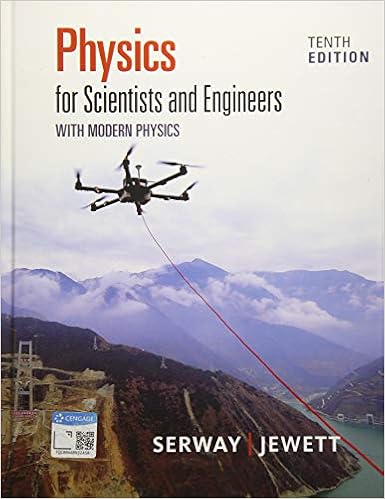# Lab Report 7 - PHYSICS I LAB EXPERIMENT SEVEN Rotational...

• Lab Report
• 7
• 83% (6) 5 out of 6 people found this document helpful

This preview shows page 1 - 3 out of 7 pages.

##### We have textbook solutions for you!
The document you are viewing contains questions related to this textbook.The document you are viewing contains questions related to this textbook.
Chapter 13 / Exercise 33
Physics for Scientists and Engineers with Modern Physics
Jewett/SerwayExpert Verified
PHYSICS I LAB EXPERIMENT SEVEN: Rotational MotionRyan Bortnick PHYS 1410L-011 (3248) June 10, 2016 Partner: Danny Stein Professor Frank Raymond Hardy
##### We have textbook solutions for you!
The document you are viewing contains questions related to this textbook.The document you are viewing contains questions related to this textbook.
Chapter 13 / Exercise 33
Physics for Scientists and Engineers with Modern Physics
Jewett/SerwayExpert Verified
Objective:The objective of this experiment was to determine the moment of inertia (also known as rotational inertia) of a free-rotational system by applying the known torque and measuring the resulting angular acceleration of the system. Data: Table 1:Data for Moment of Inertia CalculationRadius of Spindle, r = 0.0125 meterMass of Platter, m = 1.4232 kgDiameter of Platter, D = 0.217 kg(∴ Radius of Platter, R = 0.1085 meter)Idisk(=12MR2) = 8.3×10−3kg ∙ m2Angular Acceleration, I= 0.051 rad sFrictional Torque, τf= I ∝I= (8.3×10−3)(−0.051)= −4.2×10−4N ∙ mTable 2: Data for Moment of Inertia MeasurementApplied Mass 𝐦(𝐤𝐠)Angular Acceleration (?𝐚𝐝 ??)Cord Tension 𝑻 = 𝒎(?−∝ 𝒓)(𝐍)Applied Torque 𝝉 = 𝑻𝒓(𝐍 ∙ 𝐦)0.0350.4100.3434.29×10−30.0550.7520.5386.73×10−30.0751.0180.7349.18×10−30.0951.2840.9291.16×10−20.1151.5431.121.40×10−2Table 3: Changes in Torque and Moment of Inertia𝝉?= 𝑰 ∝𝑰(𝐍 ∙ 𝐦)𝒃 = ? − 𝒎?(𝐍 ∙ 𝐦)∆𝝉 =𝝉?− 𝒃𝝉?×???(%)𝒎 =??− ????− ??(𝐤𝐠 ∙ 𝐦?
•••